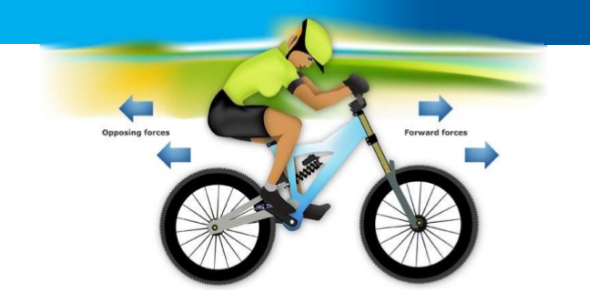# MCQ Exam On Force And Motion! Quiz

19 Questions | Total Attempts: 1288Settings.

• 1.
A book is at rest on top of a table. Which of the following is correct?
• A.

There is no force acting on the book.

• B.

The book has no inertia.

• C.

There is no force acting on the table.

• D.

The book is in equilibrium

• 2.
Which of the following situations involves friction?
• A.

A bicycle rolling down a hill

• B.

A baseball player sliding into 2nd base

• C.

A diver falling through the air to a pool

• D.

All of the above experience some friction.

• 3.
What is gravity?
• A.

Newton’s first law

• B.

The force that objects exert on each other because of their masses

• C.

The downward pull on the Earth

• D.

The friction that an object has put on it

• 4.
Which is the best example of gravity?
• A.

A car hits a tree, and its motion stops

• B.

A breeze blows, and a sailboat moves

• C.

A book is pushed, and it moves across the table

• D.

A person drops a ball, and it falls to the ground

• 5.
How does Earth ‘s gravity affect objects near  Earth?
• A.

It pushes them away.

• B.

It pulls them in

• C.

It makes them larger.

• D.

It makes them move faster.

• 6.
______________ refers to when a force is equal and opposite.
• A.

Balanced force

• B.

Unbalanced force

• C.

Magnitude

• D.

Friction

• 7.
When one force in a pair is greater than the other, we call this:
• A.

Balanced force

• B.

Unbalanced force

• C.

Magnitude

• D.

Friction

• 8.
If two forces are acting on an object they are equal in magnitude.
• A.

And equal in direction

• B.

And in opposite direction

• C.

Cancel each other

• D.

None of the above

• 9.
Which best describes the effect of force on the motion of an object?
• A.

Force causes the object to change speed or direction.

• B.

Force causes the object to experience gravity.

• C.

Force causes the object to experience balance.

• D.

Force causes the object to change shape or mass.

• 10.
What causes an object's motion to change the speed?
• A.

Forces

• B.

Volume

• C.

Mass

• D.

Shape and size

• 11.
Which example represents balanced forces?
• A.

Ball rolling down hill

• B.

Apple falling from tree

• C.

One football player is stronger than the other

• D.

When there is no changes in motion

• 12.
A type of force that pulls you towards the center of Earth is called
• A.

Friction

• B.

Push

• C.

Shove

• D.

Gravity

• 13.
A force that occurs when an object rubs against another object.
• A.

Thermometer

• B.

Conduction

• C.

Friction

• D.

• 14.
Motion is
• A.

The push or pull of an object.

• B.

A force that works against motion.

• C.

A model built by an engineer.

• D.

When an object changes position.

• 15.
The resistance caused when one object is moved in contact with another:
• A.

Force

• B.

Gravity

• C.

Friction

• D.

Wave

• 16.
When a force acts on an object, the object will usually -
• A.

Stop, move, change speed, or change direction.

• B.

Melt into a liquid and move.

• C.

Create nuclear energy.

• D.

Evaporate into air.

• 17.
Which of the following CANNOT occur as a result of unbalanced forces?
• A.

The object's motion can speed up

• B.

The object's motion can slow down

• C.

The object's motion can stay the same

• D.

The object's motion can stop

• 18.
What force causes a teardrop to roll down someone's cheek?
• A.

Friction

• B.

Magnetism

• C.

Gravity

• D.

Acceleration

• 19.
If two arm wrestlers exert a force on each other's hands and the hands don't move, what can we say about the force between the two hands?
• A.

The forces must be weak

• B.

The forces must be unbalanced

• C.

The forces must be strong

• D.

The forces must be balanced

Related TopicsBack to top